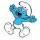Home
IT Knowledge
Inspiration
Languages
EN

# Java 8 - create stream

0 points
Created by:rmlocerd
212

In this article, we would like to show you how to create a stream in Java 8.

Quick solution:

``````Collection<String> collection = Arrays.asList("A", "B", "C");

// Create stream from the collection
Stream<String> stream = collection.stream();``````

## 1. Create a stream from Collection

In this example, we present how to create a stream from any type of Collection such as Collection, List or Set.

``````import java.util.*;
import java.util.stream.Stream;

public class Example {

public static void main(String[] args) {
Collection<String> collection = Arrays.asList("A", "B", "C");

// Create stream from the collection
Stream<String> streamFromCollection = collection.stream();

// print elements
streamFromCollection.forEach(System.out::println);
}
}``````

Output:

``````A
B
C``````

## 2. Create a stream from specified values

In this section, we present how to create a stream from specified values such as Strings or Integers.

### 2.1 String values

``````import java.util.stream.Stream;

public class Example {

public static void main(String[] args) {
Stream<String> streamOfLetters = Stream.of("A", "B", "C");

// print elements
streamOfLetters.forEach(System.out::println);
}
}``````

Output:

``````A
B
C``````

### 2.2 Integer values

``````import java.util.stream.Stream;

public class Example {

public static void main(String[] args) {
Stream<Integer> streamOfLetters = Stream.of(1, 2, 3);

// print elements
streamOfLetters.forEach(System.out::println);
}
}``````

Output:

``````1
2
3``````

## 3. Create a stream from an array

### 3.1 Create a stream from the whole array

In this example, we present how to create a stream from an array using `Arrays.stream()` method.

``````import java.util.Arrays;
import java.util.stream.Stream;

public class Example {

public static void main(String[] args) {
String[] letters = new String[]{"A", "B", "C", "D"};
Stream<String> streamOfArray = Arrays.stream(letters);

// print elements
streamOfArray.forEach(System.out::println);
}
}``````

Output:

``````A
B
C
D``````

### 3.2 Create a stream from part of the array

In this example, we present how to create a stream from part of the array using `Arrays.stream()` with the following syntax:

``Arrays.stream(array, indexStart, indexEnd);``

Practical example:

``````import java.util.Arrays;
import java.util.stream.Stream;

public class Example {

public static void main(String[] args) {
String[] letters = new String[]{"A", "B", "C", "D"};
Stream<String> streamOfArray = Arrays.stream(letters, 0, 2);

// print elements
streamOfArray.forEach(System.out::println);
}
}``````

Output:

``````A
B``````